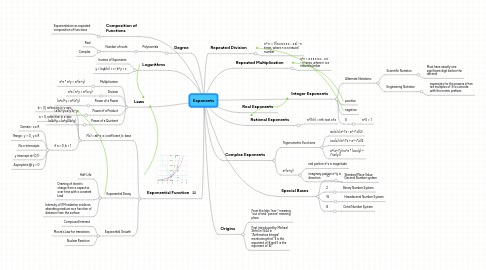# Exponents

Get Started. It's FreeExponents## 1. Degree

### 1.1. Polynomials

1.1.1. Number of roots

1.1.1.1. Real

1.1.1.2. Complex

## 3. Laws

### 3.1. Multiplication

3.1.1. a^x * a^y = a^(x+y)

### 3.2. Division

3.2.1. a^x / a^y = a^(x-y)

### 3.3. Power of a Power

3.3.1. (a^x)^y = a^(x*y)

### 3.4. Power of a Product

3.4.1. (a*b)^y=a^y*b^y

### 3.5. Power of a Quotient

3.5.1. (a/b)^y = (a^y)/(b^y)

## 4. Exponential Function

### 4.1. F(x) = ab^x, a: coefficient, b: base

4.1.1. b < |1|, reflection in y-axis

4.1.2. a < 0, reflection in x-axis

4.1.3. if a > 0, b > 1

4.1.3.1. Domain : x e R

4.1.3.2. Range : y > 0 , y e R

4.1.3.3. No x-intercepts

4.1.3.4. y intercept at (0,1)

4.1.3.5. Asymptote @ y = 0

### 4.2. Exponential Decay

4.2.1. Half-Life

4.2.2. Draining of electric charge from a capacitor over time with a constant load

4.2.3. Intensity of EM radiation inside an absorbing medium as a function of distance from the surface

### 4.3. Exponential Growth

4.3.1. Compound Interest

4.3.2. Moore's Law for transistors

4.3.3. Nuclear Reaction

## 7. Integer Exponents

### 7.1. Alternate Notations

7.1.1. Scientific Notation

7.1.1.1. Must have exactly one significant digit before the decimal

7.1.2. Engineering Notation

7.1.2.1. exponents for the powers of ten are multiples of 3 to coincide with the metric prefixes

7.4.1. a^0 = 1

## 9. Complex Exponents

### 9.1. Trigonometric Functions

9.1.1. sin(z)=(e^i*z - e^-i*z)/2i

9.1.2. cos(z)=)e^i*z + e^-i*z)/2

9.1.3. e^(x+i*y)=e^x * (cos(y) + i*sin(y))

### 9.2. e^(x+iy)

9.2.1. real portion e^x is magnitude

9.2.2. imaginary portion e^iy is direction

## 13. Special Bases

### 13.1. 10

13.1.1. Standard Place Value; Decimal Number system

### 13.2. 2

13.2.1. Binary Number System